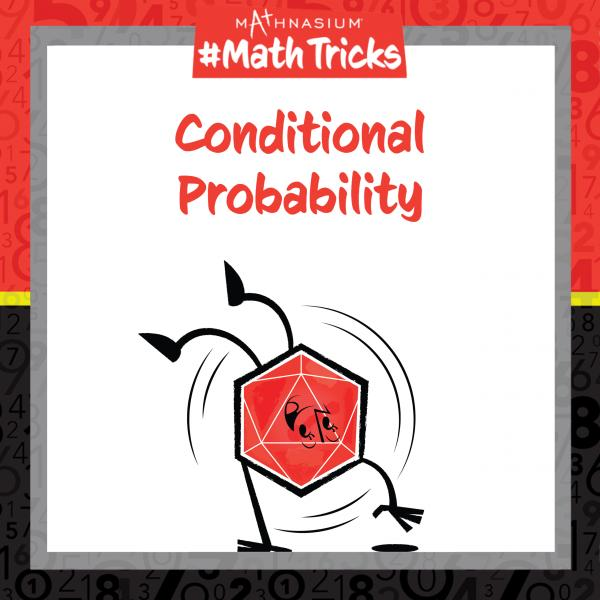# Mathnasium #MathTricks: Probability (Part 3)

By Mathnasium | Added Apr 26, 2023Welcome to Mathnasium’s Math Tricks series. Today we are calculating the conditional probability of one event occurring, given that another has already occurred.

Two events are considered dependent if the occurrence of one event affects the probability of the other event. For example, if we draw a red marble from a bag of multicoloured marbles and do not replace it, the probabilities of drawing different coloured marbles change; so, drawing marbles of particular colours from a bag without replacing them are dependent events.

The probability of a dependent event, B, occurring given that another event, A, has already occurred is called a conditional probability, and is denoted as: P(B | A). Conditional probabilities can change based on some event that has already occurred.

Follow the example below to find the conditional probability.

##### Example: A jar contains 8 quarters, 6 dimes, and 10 pennies. If a quarter is removed from the jar and not replaced, what is the probability of then randomly drawing another quarter?

Step 1: Identify the two dependent events.

The two dependent events are “drawing a quarter” and “drawing a quarter.” We want to find the probability of drawing a quarter given that we have already drawn one quarter from the jar:
P(quarter | quarter), or P(Q | Q).

Step 2: Find the probability of the first dependent event.
The probability of drawing a quarter is: P(Q) = 824 = 13.

Step 3: Find the conditional probability of the second event occurring, given that the first event occurred.
The probability of drawing a quarter, given that you already drew a quarter is: P(Q | Q) = 723.